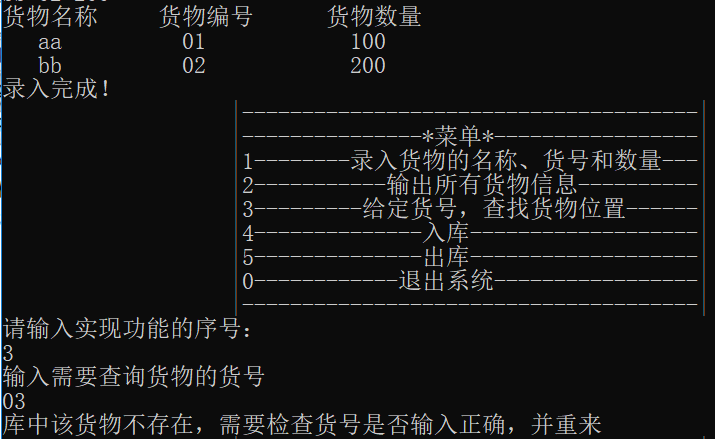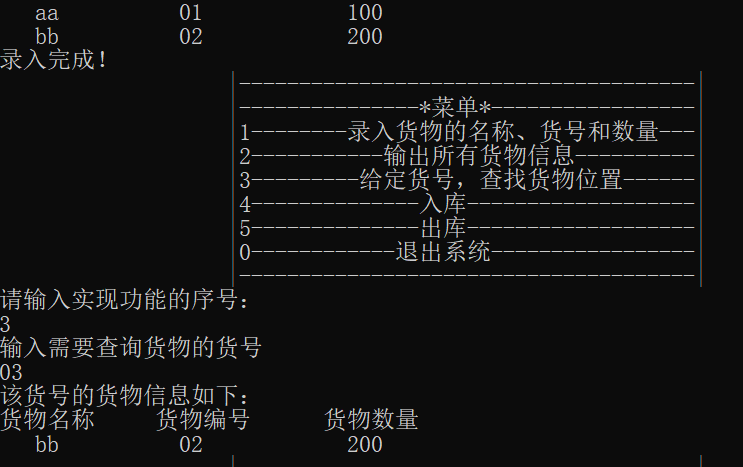# 数据结构C语言链表完成一个货物信息管理系统

*/*货物信息：名称，货号，数量

1--------录入所有货物名称、货号、数量
2---------输出所有货物信息
3---------给定货号，查找货物位置
4---------入库
5----------出库
*/

#include <stdio.h>
#include <stdlib.h>
#include <string.h>
typedef struct
{
char name,     //货物名称（巧妙的运用了逗号运算符）
no;       //货物编号
int num;           //货物数量
}good;                //定义货物类型的结构体
typedef struct  node     //节点类型的结构体
{
good data;         //货物数据域
struct  node *next;  //存放下一个货物数据的地址，即指针域
}LNode;
//菜单函数
{
printf("%60s","|--------------------------------------|\n");
printf("%60s","|---------------*菜单*-----------------|\n");
printf("%60s","|1--------录入货物的名称、货号和数量---|\n");
printf("%60s","|2-----------输出所有货物信息----------|\n");
printf("%60s","|3---------给定货号，查找货物位置------|\n");
printf("%60s","|4--------------入库-------------------|\n");
printf("%60s","|5--------------出库-------------------|\n");
printf("%60s","|0------------退出系统-----------------|\n");
printf("%60s","|--------------------------------------|\n");
}
//录入基本信息
//定义一个返回类型为结点类型的函数，因为要返回头指针，方便主函数进行其他操作
{
LNode *s,*r;
printf("输入货物个数：\n");
int i,len;
good x;
scanf("%d",&len);
printf("输入货物名称、货物编号和数量：\n");
for(i=1;i<=len;i++){
scanf("%s%s%d",x.name,x.no,&x.num);
s=(LNode*)malloc(sizeof(LNode));
s->data=x;
s->next=NULL;
r->next=s;
r=s;
}
}
//输出所有货物信息
{
printf("货物名称     货物编号      货物数量\n");
while(p!=NULL)
{
printf("%5s%12s%15d\n",p->data.name,p->data.no,p->data.num);
p=p->next;
}
}
//根据货号查找货物信息
{
while((p!=NULL)&&strcmp(p->data.no,x.no)!=0)
p=p->next;
return p;
}
/*入库函数   过程：
先查找，若存在，修改数量；否则，按货物号有序插入*/
{

int In_Num;
LNode *k,
printf("输入需要入库的货号:\n");
scanf("%s",x.no);
good y=x;
while((p!=NULL)&&strcmp(p->data.no,x.no)!=0)
p=p->next;
if(p==NULL)
{
printf("该货物不存在，需录入该货物信息，并以编号有序插入\n");
printf("请输入货物名称、货号和数量\n");
scanf("%s%s%d",x.name,x.no,&(x.num));
if(strcmp(x.no,y.no)!=0)
printf("输入货号与查询货号不一致,重来\n");
else
{
k=(LNode *)malloc(sizeof(LNode));
while(r->next!=NULL&&strcmp(r->next->data.no,x.no)<0)
r=r->next;
k->data=x;
k->next=r->next;
r->next=k;
printf("显示入库更新结果:\n");
printf("货物名称     货物编号      货物数量\n");
printf("%5s%12s%15d\n",k->data.name,k->data.no,k->data.num);
printf("输出出库后库存信息:\n");
}
}
else
{
printf("请输入入库数量：\n");
scanf("%d",&In_Num);
printf("显示入库结果:\n");
p->data.num+=In_Num;
printf("货物名称     货物编号      货物数量\n");
printf("%5s%12s%15d\n",p->data.name,p->data.no,p->data.num);
printf("输出出库后库存信息\n");
}
}
/*出库函数   过程如下：
先查找，若存在，分三种情况：
库存数量有多余：修改数量
库存数量不足：需要用户确认
库存数量刚好：删除
若不存在，给出提示*/
{
int Out_Num;
LNode *k;
if(k==NULL)
printf("不存在此编号货物\n");
else
{
printf("请输入出库数量：\n");
scanf("%d",&Out_Num);
if(k->data.num<Out_Num)
printf("输入出库数量错误，请用户确认是否输入正确\n");
else
{
if(k->data.num>Out_Num)
k->data.num-=Out_Num;
else
if(k->data.num==Out_Num)//如果出库数等于原有货物数量，清除该货物
{
while(q->next!=k)
q=q->next;
q->next=k->next;//k元素前面的节点的地址指向k后面节点的地址
free(k);  //释放k节点
printf("该货物全部出库，已删除\n");
}
printf("输出出库后剩余货物信息\n");
printf("出库成功！\n");

}
}
}
int main()
{
good x;
int sel;
LNode *k;
do
{
printf("请输入实现功能的序号：\n");
scanf("%d",&sel);
switch(sel)
{
printf("录入完成！\n");
break;
case 2: printf("输出已有货物名称、货号和数量\n");
case 3: printf("输入需要查询货物的货号\n");
scanf("%s",x.no);
if(k==NULL)
printf("库中该货物不存在，需要检查货号是否输入正确，并重来\n");
else
{
printf("该货号的货物信息如下：\n");
printf("货物名称     货物编号      货物数量\n");
printf("%5s%12s%15d\n",k->data.name,k->data.no,k->data.num);
}
break;
break;
case 5:printf("请输入出库货号：\n");
scanf("%s",x.no);
break;
default:break;
}
}while(sel!=0);
return 0;
}


case1：input，即把信息输入到链表中

 LNode *input(LNode *head)
//定义一个返回类型为结点类型的函数，因为要返回头指针，方便主函数进行其他操作
{
LNode *s,*r;                     //存储数据的节点
int i,len;                           //控制循环输入
good x;                           //定义一个货物类型，以便输入数据
printf("输入货物个数：\n");
scanf("%d",&len);
printf("输入货物名称、货物编号和数量：\n");//以下即为尾插法实现数据信息的录入
for(i=1;i<=len;i++){
scanf("%s%s%d",x.name,x.no,&x.num);
s=(LNode*)malloc(sizeof(LNode));     //为新结点申请一份空间
s->data=x;            //把数据存入节点的数据域中
s->next=NULL;    //置待插入的节点s为链尾节点
r->next=s;            //在链尾插入s节点，即r的指针域存放s节点的指针
r=s;                    //r后移，使r指向新的链尾节点s
}
}


//这里就不需要r=head;
for(i=1;i<=len;i++){
scanf("%s%s%d",x.name,x.no,&x.num);
s=(LNode*)malloc(sizeof(LNode));     //为新结点申请一份空间
s->data=x;            //把数据存入节点的数据域中
}


    r=head;                                   //r始终指向链尾节点，因为head原本指的是尾结点
for(i=1;i<=len;i++){
scanf("%s%s%d",x.name,x.no,&x.num);
s=(LNode*)malloc(sizeof(LNode));     //为新结点申请一份空间
s->data=x;            //把数据存入节点的数据域中
s->next=NULL;    //置待插入的节点s为链尾节点
r->next=s;            //在链尾插入s节点，即r的指针域存放s节点的指针
r=s;                    //r后移，使r指向新的链尾节点s
}


case 2:print() 输出 把库存信息输出

//输出所有货物信息
{
printf("货物名称     货物编号      货物数量\n");
while(p!=NULL)
{
printf("%5s%12s%15d\n",p->data.name,p->data.no,p->data.num);
p=p->next;
}
}


case3:search 查找 按值查找

//根据货号查找货物信息
{
while((p!=NULL)&&strcmp(p->data.no,x.no)!=0)
p=p->next;
return p;
}LNode* search(LNode *head,good x)
{
while((p->next!=NULL)&&strcmp(p->data.no,x.no)!=0)
p=p->next;
return p;
}case4:getin(); 入库

/*入库函数   过程：
先查找，若存在，修改数量；否则，按货物号有序插入*/
{

int In_Num;              //定义入库数量
LNode *k,               //存放重新录入的货物的信息，以便后期插入
printf("输入需要入库的货号:\n");
scanf("%s",x.no);
good y=x;
while((p!=NULL)&&strcmp(p->data.no,x.no)!=0)
p=p->next;
if(p==NULL)
{
printf("该货物不存在，需录入该货物信息，并以编号有序插入\n");
printf("请输入货物名称、货号和数量\n");
scanf("%s%s%d",x.name,x.no,&(x.num));
if(strcmp(x.no,y.no)!=0)                   /*以防输入编号时候和之前不存在要录入的编号不相同，给以提示*/
printf("输入货号与查询货号不一致,重来\n");
else
{
k=(LNode *)malloc(sizeof(LNode));
while(r->next!=NULL&&strcmp(r->next->data.no,x.no)<0)
r=r->next;
k->data=x;
k->next=r->next;     //插入操作，注意代码顺序，以防节点自己指向自己，导致断链
r->next=k;
printf("显示入库更新结果:\n");
printf("货物名称     货物编号      货物数量\n");
printf("%5s%12s%15d\n",k->data.name,k->data.no,k->data.num);
printf("输出出库后库存信息\n");
}
}
else
{
printf("请输入入库数量：\n");
scanf("%d",&In_Num);
printf("显示入库结果\n");
p->data.num+=In_Num;
printf("货物名称     货物编号      货物数量\n");
printf("%5s%12s%15d\n",p->data.name,p->data.no,p->data.num);
printf("输出出库后库存信息\n");
}
}


                    k->next=r->next;
r->next=k;


case 5:getout(); 出库

/*出库函数   过程如下：
先查找，若存在，分三种情况：
库存数量有多余：修改数量
库存数量不足：需要用户确认
库存数量刚好：删除
若不存在，给出提示*/
{
int Out_Num;
LNode *k;
if(k==NULL)
printf("不存在此编号货物\n");
else
{
printf("请输入出库数量：\n");
scanf("%d",&Out_Num);
if(k->data.num<Out_Num)     //出库数量大于已有的库存
printf("输入出库数量错误，请用户确认是否输入正确\n");
else
{
if(k->data.num>Out_Num)     //正常出库
k->data.num-=Out_Num;
else
if(k->data.num==Out_Num)//如果出库数等于原有货物数量，清除该货物
{
while(q->next!=k)
q=q->next;      //查找到k节点的前一个节点
q->next=k->next; //k元素前面的节点的地址指向k后面节点的地址，即可完成删除操作
free(k);  //释放k节点内存
printf("该货物全部出库，已删除\n");
}
printf("输出出库后剩余货物信息\n");
printf("出库成功！\n");

}
}
}


 while(q->next!=k->next)
q=q->next;


 while(q->next!=k)
q=q->next;


01-0706-144178
07-0559
10-132632
05-183233
09-161594
03-04
08-14649
06-061万+
01-042万+
06-153721
11-021819
02-161542
11-021046
01-021255
05-1849
04-052378点击重新获取扫码支付余额充值